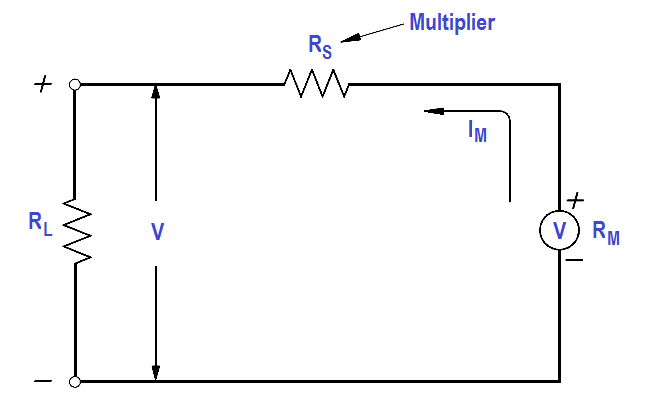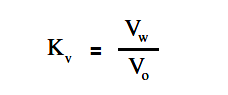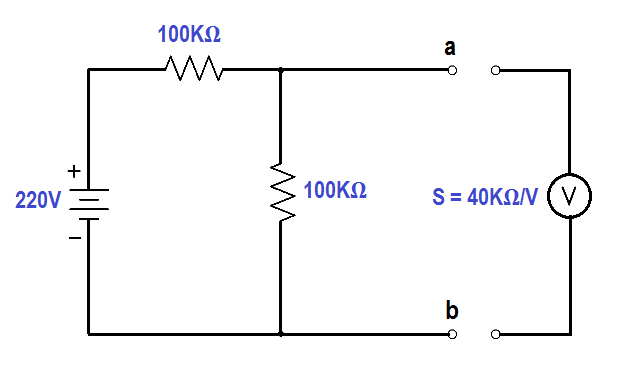# Voltmeters

A simple DC voltmeter can be constructed by placing a resistor (RS), called a multiplier, in series with the ammeter meter movement, and marking the meter face to read voltage (as shown in Figure).

Voltmeters are connected in parallel with the load (RL) being measured.Figure : Simple DC Voltmeter

When constructing a voltmeter, the resistance of the multiplier must be determined to measure the desired voltage. The Equation is a mathematical representation of the voltmeter’s multiplier resistance.

##### ImRs = V – ImRmwhere

V = voltage range desired
I= meter current
Rm = meter resistance
Rs = multiplier resistance or series resistance

Example:

A 2 mA meter movement with internal resistance of 25 ohms is to be constructed as a voltmeter. What value must the series resistance be to measure full scale voltage of 100 volts?

Solution :Since Rm is negligibly low, then

Rs = V/Im

Rs = 100 / (2×10-3)

Rs =  50kΩ

When a voltmeter is connected in a circuit, the voltmeter will draw current from that circuit. This current causes a voltage drop across the resistance of the meter, which is subtracted from the voltage being measured by the meter. This reduction in voltage is known as the loading effect and can have a serious effect on measurement accuracy, especially for low current circuits.

The accuracy of a voltmeter (Kv ) is defined as the ratio of measured voltage when the meter is in the circuit (Vw) to the voltage measured with the meter out of the circuit.

The below Equation is a mathematical representation of the accuracy of a voltmeter, or true voltage (Vo).Meter accuracy can also be determined by comparing the relationship between the input and circuit resistances using Ohm’s Law as described below.where

Im = meter current
Vo = true voltage
Ro = circuit resistance
Rin = input resistance of the voltmeter
Vw = indicated voltage
Kv = meter accuracy

Example:

A voltmeter in the 100 volt range with a sensitivity of 40 KΩ/V is to measure the voltage across terminals ab.Find :

1. Vo , true voltage
2. Vw , indicated voltage
3. Kv , meter accuracy

Solution :

Find Vo , true voltage

Vo =  { 100 KΩ / (100 KΩ + 100 KΩ) } x 220 volts

Vo = 110 volts

Find Vw , indicated voltage

R= (100 x 100) / (100 + 100) = 50KΩ

Rin = S V = 40KΩ x 100 = 4.4 MΩ

V= Rin / (Ro + Rin )

V= { 4.4MΩ / (50KΩ + 4.4MΩ) } 110 volts

V= 108.9 volts

Find Kv , meter accuracy

Kv = V/ Vo

Kv = 108.9/110

Kv = 0.99 or 99%